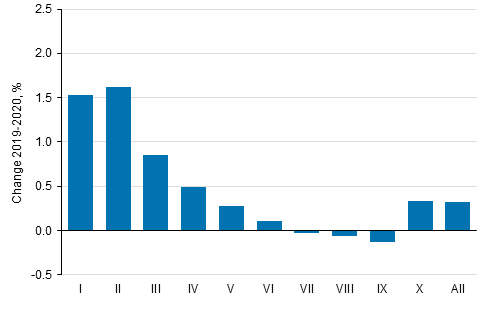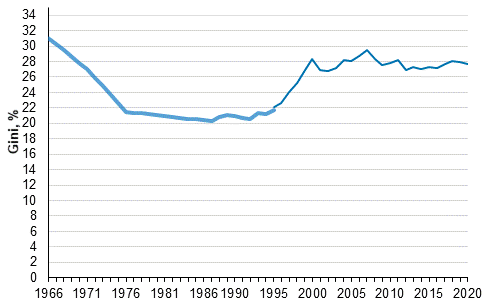Data published after 5 April 2022 can be found on the renewed website.

Go to the new statistics page

Published: 15 December 2021

# Income differentials decreased slightly in 2020

Statistics Finland's income distribution statistics show that income differentials decreased slightly in 2020 compared with the previous year. Real income increased by 1.5 per cent in two lowest-income deciles from 2019 to 2020. The income level of the population's highest-income decile increased by 0.3 per cent. The income of medium income earners (income deciles V to IX) remained at the previous year’s level.

Change in average real income (%) by income decile from 2019 to 2020Income decile: the population is arranged according to income and divided into ten equal parts, each of which has around half a million persons. The first (I) income decile is the lowest-income decile and the tenth (X) is the highest-income decile. Income concept: equivalent disposable monetary income (incl. realised capital gains) of household dwelling-unit, average per person. Equivalent income: the household-dwelling unit’s income divided by its consumption units (modified OECD-scale).

In 2020, the Gini coefficient describing relative income differentials received the value 27.7, which was 0.2 percentage points lower than in the previous year and 0.2 percentage lower than in 2010. Compared with 1995, the Gini coefficient has grown by around 5.5 percentage points. Most of this is caused by the fast growth of income differentials at the end of the 1990s. The income differentials have been at their lowest in the mid-1980s. The Gini coefficient gets the value 0 if everyone receives the same amount of income and 100 if one income earner receives all the income. The higher the Gini coefficient, the bigger the relative income differentials are.

Development of income inequality 1966–2020, Gini coefficient (%)Persons belonging to the household-dwelling population, the household-dwelling unit's equivalent disposable monetary income (incl. realised capital gains). Sources: 1995 to 2020 Income distribution statistics’ total data; 1986 to 1995: Income distribution statistics’ sample data; 1966,1971,1976,1981 Household Budget Survey. The missing intervening years (1967 to 1985) have been linearly interpolated.

Source: Income distribution statistics 2020, Statistics Finland

Inquiries: Usva Topo 029 551 3036, Pekka Ruotsalainen 029 551 2610, Kaisa-Mari Okkonen 029 551 3408, toimeentulo.tilastokeskus@stat.fi

Head of Department in charge: Hannele Orjala

Tables

Tables in databases

Pick the data you need into tables, view the data as graphs, or download the data for your use.

Updated 15.12.2021

Referencing instructions:

Official Statistics of Finland (OSF): Income distribution statistics [e-publication].
ISSN=1799-1331. Income inequality (international comparison) 2020. Helsinki: Statistics Finland [referred: 29.5.2023].
Access method: http://www.stat.fi/til/tjt/2020/01/tjt_2020_01_2021-12-15_tie_001_en.html